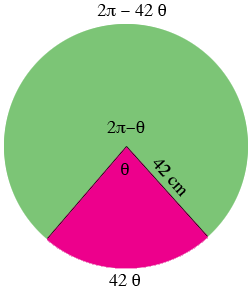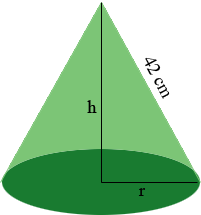SEARCH HOMEMath Central Quandaries & QueriesQuestion from Ella: Hello, this is question - 'If you take a circle with a radius of 42cm and cut a sector from it, the remaining shape can be curled around to form a cone. Find the sector angle that produces the maximum volume for the cone made from your circle.'Hi Ella,

In my diagram the sector shaded red is cut out and removed and the remaining sector, shaded green, is used to form the cone. The sector angle of the red sector measures $\theta$ radians an thus the sector angle of the green sector measures $2 \pi - \theta$ radians. The length of the arc that borders the green sector has length $2 \pi - 42 \theta$ cm.The cone formed has volume $V = \large \frac{1}{3} \normalsize \pi r^2 h$ cubic centimeters using the notation in the diagram below. This expression is a function of $r$ and $h$ but if you can express $r$ and $h$ in terms of $\theta$ you can use the calculus you know to maximize $V$ as a function of $\theta.$The dark green base is a circle of radius $r$ and its circumference is the arc that borders the green sector has length $2 \pi - 42 \theta$ cm in the first diagram. The circumference if a circle of radius $r$ cm is $2 \pi r$ cm and hence you can express $r$ in terms of $\theta.$ The triangle with sides of length $r, h$ and 42 cm in the diagram above is a fight triangle and hence you can use Pythagoras Theorem to express $h$ in terms of $r$ and hence in terms of $\theta.$

I hope this helps,
PennyMath Central is supported by the University of Regina and The Pacific Institute for the Mathematical Sciences.